Courses
Courses for Kids
Free study material
Free LIVE classes
More

# Division Word ProblemsLIVE
Join Vedantu’s FREE Mastercalss

## Overview of Division

Small numbers can be divided into groups and pieces using the simple division method in mathematics. Divide easy and simple division problems into terms that can be divided using simple division sums.

One of the four fundamental mathematical operations, along with addition, subtraction, and multiplication, is division. Division is the process of dividing a larger group into smaller groups so that each group contains an equal number of things. In this article, you will get some maths word problems, especially division problems and you will also learn how to solve these division problems.

## Division Problem

A division problem has three primary components: the dividend, the divisor, and the quotient. The amount that will be divided is the dividend. The quantity of "people" among whom the number is divided is referred to as the divisor. The answer is the quotient.

Other than these 3 components, the remainder is also one component of division. In the case when a dividend is completely divided by the divisor, the remainder will be zero and in other cases, it will be more than zero.

For example, if we divide 100 by 8, we get a remainder greater than 0 i.e., 4.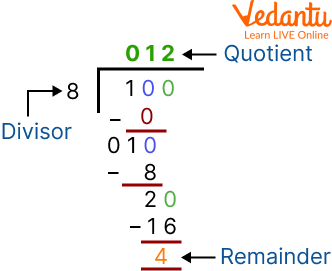Dividing 100 by 8

If we divide 300 by 100 then the remainder is zero.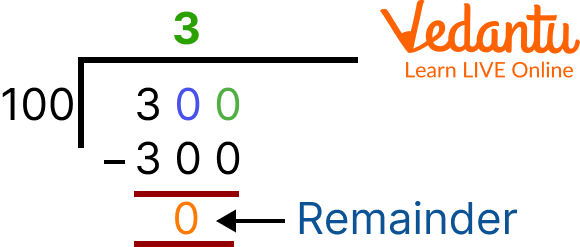Dividing 300 by 100

## How to Write Division in Word

A division symbol (÷) is used to show division. You must have seen this symbol in your maths book, otherwise, if you don’t insert a dividing symbol then the whole division problem can easily be written in words. Like dividing 50 by 5, in this way division is written in words without inserting symbols.

Now, we will see the steps of division. These steps will help you to understand how division is performed.

## Steps of Division

Step 1: The Problem should be written out using a Long Division Bar.

The division bar ( 厂 ) resembles a pair of closing parenthesis joined to a horizontal line that crosses the row of numbers directly below the bar. Put the dividend, which is the number you'll be dividing into, inside the long division bar and the divisor, which is the number you'll be dividing into, outside.

For example, divide 65 by 5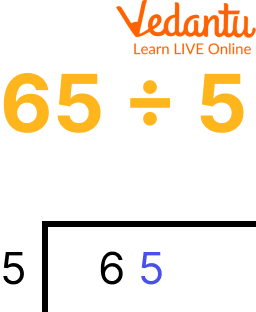Step 1

Step 2: Divide the Divisor by the Dividend's Leading Digit.

Calculate how many times the divisor (the number outside the division bar) enters the dividend's first digit. Put the entire number result directly over the first digit of the divisor, above the division line.Step 2

Step 3: Divide the Divisor by the Number above the Division Bar.

Multiply the amount you just put above the division bar by the denominator (the number to the left of the division bar). Under the dividend, in a new row, align the result with the dividend's first digit.Step 3

Step 4: Subtract the Multiplication Outcome from the Dividend's First Digit.

Alternatively stated, divide the sum you just entered into the new row beneath the dividend by the digit in the dividend immediately above it. The digits from the subtraction issue should be aligned with the result in a new row.Step 4

Step 5: Bring the Dividend's Second Digit Down.

Just to the right of the result of your recent subtraction, move the second digit of the dividend down to the new bottom row.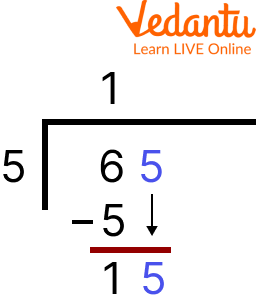Step 5

Step 6: The Long Division Procedure should be Repeated.

Use the dividend (the number in the division bar to the left) and the new number in the bottom row this time (the result of your first round of calculations and the digit you carried down). As previously, divide, multiply, and then subtract to determine your outcome.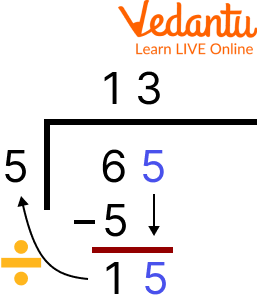Step 6

Step 7: Carry out Long Division once more.

As previously, you begin by dividing, move on to multiplying, and end by subtracting. Do another round of division and get the remainder.

Here are some division word problems that are shared. These are solved examples.

1. Rs. 670 are distributed equally among 7 men. How much money will each person get?
Ans: Money received by 7 men = 670

So, money received by one man = 670 ÷ 7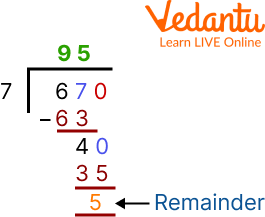Dividing 670 by 7

670 ÷ 7 = 95

Therefore, each person gets Rs. 95

2. An active dolphin needs to breathe 7 times a minute. How long would it take them to breathe 49 times?
Ans: Dolphin needs to breathe 7 times a minute.

Time is taken by a dolphin to breathe 49 times = 49 ÷ 7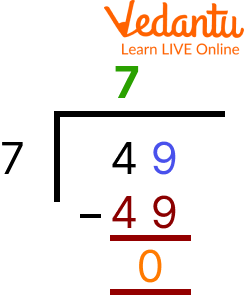Dividing 49 by 7

So, 49 ÷ 7 = 7, It would take 7 minutes.

3. Jason has 406 socks in total in his shop which he puts into pairs. How many pairs of socks does he have?
Ans: Total number of socks Jason has in pairs = 406

Number of paired socks = 406 ÷ 7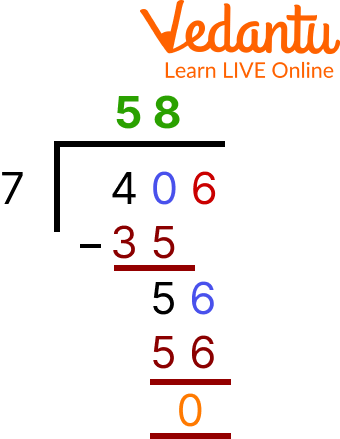Dividing 406 by 7

So, 406 ÷ 7 = 58, She owns 58 pairs of socks.

## Practise Questions

Q 1. Jasmine runs 3 metres in a minute. How long will it take for her to run 27 metres at the same speed?
Ans: It would take her 9 minutes.

Q 2. An episode of Sally's favourite cartoon runs for 10 minutes. How many episodes can she watch in 1 hour?
Ans: She can watch 6 episodes.

## Summary

In this article, firstly division and important parts of division i.e., divisor, dividend, quotient, and the remainder are discussed. Steps of division for kids are discussed by using examples and pictures of the same. There are 7 main steps present in this division part. Steps of division are applied in a division word problem. Some maths word problems of division are mentioned with proper explanation. Daily life examples are shared in the division word problem.

Last updated date: 27th Sep 2023
Total views: 111.6k
Views today: 3.11k

## FAQs on Division Word Problems

1. What is a long division method?

Long division method is one way to divide a big, multi-digit number into another big, multi-digit number by using steps of division and operations of multiplication, subtraction and division.

2. What is the division formula?

The division of two numbers can be calculated using the following formula: Dividend = Divisor x Quotient + Remainder. The number that is being divided in this case is known as the dividend. The number that divides the number (dividend) into equal parts is known as the divisor.

3. What is the importance of division and how is it used in real life?

The abilities your child has already mastered in addition, subtraction, and multiplication are built upon by division. Students will study long division with multi-digit numbers and remainders after mastering the fundamentals of division.

Example of Division in Real Life:

She purchases a bag containing 340 pieces of candy. For a forthcoming holiday, she wants to divide up the candy into treat bags for her students. How many pieces should be given to each kid? Considering that a teacher will undoubtedly assign equal numbers, the student must divide.